# 内存泄漏-原因、避免和定位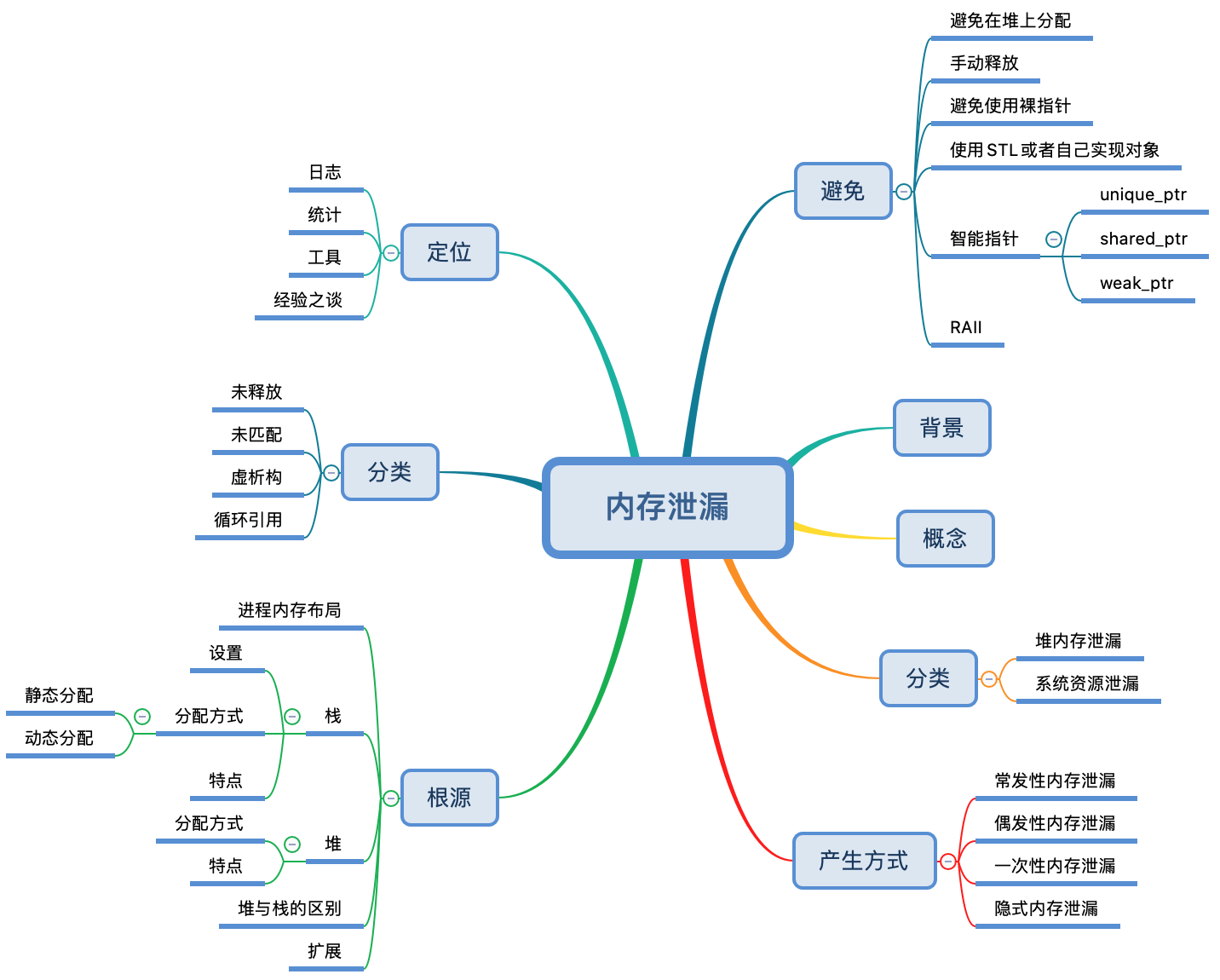## 背景

C/C++语言中，内存的分配与回收都是由开发人员在编写代码时主动完成的，好处是内存管理的开销较小，程序拥有更高的执行效率；弊端是依赖于开发者的水平，随着代码规模的扩大，极容易遗漏释放内存的步骤，或者一些不规范的编程可能会使程序具有安全隐患。如果对内存管理不当，可能导致程序中存在内存缺陷，甚至会在运行时产生内存故障错误。

## 概念## 分类

• 堆内存泄漏：我们经常说的内存泄漏就是堆内存泄漏，在堆上申请了资源，在结束使用的时候，没有释放归还给OS，从而导致该块内存永远不会被再次使用
• 资源泄漏：通常指的是系统资源，比如socket，文件描述符等，因为这些在系统中都是有限制的，如果创建了而不归还，久而久之，就会耗尽资源，导致其他程序不可用

## 根源

### 进程内存布局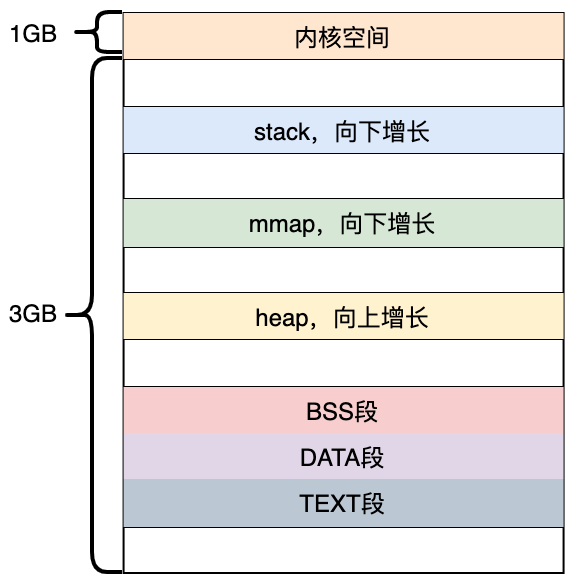• 内核空间：供内核使用，存放的是内核代码和数据
• stack：这就是我们经常所说的栈，用来存储自动变量(automatic variable)
• mmap:也成为内存映射，用来在进程虚拟内存地址空间中分配地址空间，创建和物理内存的映射关系
• heap:就是我们常说的堆，动态内存的分配都是在堆上
• bss:包含所有未初始化的全局和静态变量，此段中的所有变量都由0或者空指针初始化，程序加载器在加载程序时为BSS段分配内存
• ds:初始化的数据块
• 包含显式初始化的全局变量和静态变量
• 此段的大小由程序源代码中值的大小决定，在运行时不会更改
• 它具有读写权限，因此可以在运行时更改此段的变量值
• 该段可进一步分为初始化只读区和初始化读写区
• text：也称为文本段
• 该段包含已编译程序的二进制文件。
• 该段是一个只读段，用于防止程序被意外修改
• 该段是可共享的，因此对于文本编辑器等频繁执行的程序，内存中只需要一个副本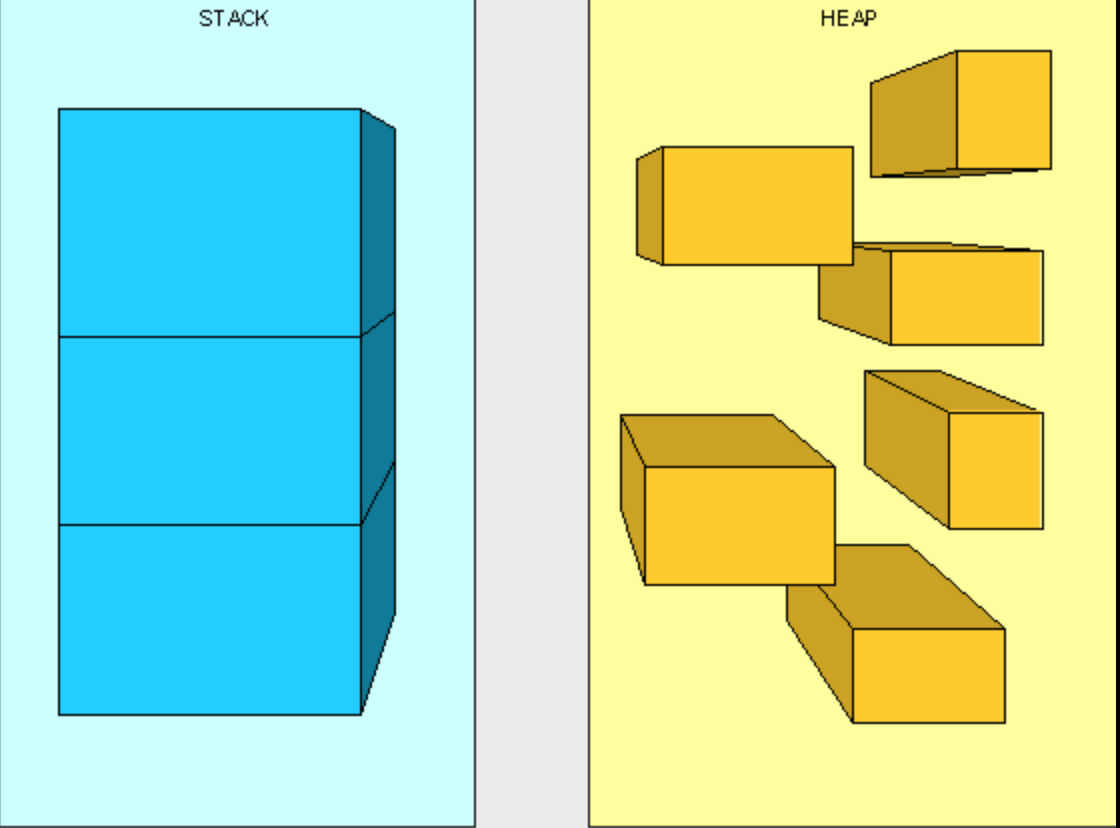### 栈

class Object {
public:
Object() = default;
// ....
};

void fun() {
Object obj;

// do sth
}


#### 设置

ulimit -s
10240


ulimit -s 102400


/etc/security/limits.conf


#### 分配方式

##### 静态分配

void fun() {
int a;
}


#### 特点

• 分配速度快：分配大小由编译器在编译器完成
• 不会产生内存碎片：栈内存分配是连续的，以FIFO的方式进栈和出栈
• 大小受限：栈的大小依赖于操作系统
• 访问受限：只能在当前函数或者作用域内进行访问

### 堆

#### 特点

• 变量可以在进程范围内访问，即进程内的所有线程都可以访问该变量
• 没有内存大小限制，这个其实是相对的，只是相对于栈大小来说没有限制，其实最终还是受限于RAM
• 相对栈来说访问比较慢
• 内存碎片
• 由开发者管理内存，即内存的申请和释放都由开发人员来操作

### 堆与栈区别

• 空间大小不同
• 一般来讲在 32 位系统下，堆内存可以达到4G的空间，从这个角度来看堆内存几乎是没有什么限制的。
• 对于栈来讲，一般都是有一定的空间大小的，一般依赖于操作系统(也可以人工设置)
• 能否产生碎片不同
• 对于堆来讲，频繁的内存分配和释放势必会造成内存空间的不连续，从而造成大量的碎片，使程序效率降低。
• 对于栈来讲，内存都是连续的，申请和释放都是指令移动，类似于数据结构中的进栈和出栈
• 增长方向不同
• 对于堆来讲，生长方向是向上的，也就是向着内存地址增加的方向
• 对于栈来讲，它的生长方向是向下的，是向着内存地址减小的方向增长
• 分配方式不同
• 堆都是动态分配的，比如我们常见的malloc/new；而栈则有静态分配和动态分配两种。
• 静态分配是编译器完成的，比如局部变量的分配，而栈的动态分配则通过alloca()函数完成
• 二者动态分配是不同的，栈的动态分配的内存由编译器进行释放，而堆上的动态分配的内存则必须由开发人自行释放
• 分配效率不同
• 栈有操作系统分配专门的寄存器存放栈的地址，压栈出栈都有专门的指令执行，这就决定了栈的效率比较高
• 堆内存的申请和释放专门有运行时库提供的函数，里面涉及复杂的逻辑，申请和释放效率低于栈

### 扩展

#include <stdio.h>
#include <stdlib.h>

int main() {
int a;
int *p;
p = (int *)malloc(sizeof(int));
free(p);

return 0;
}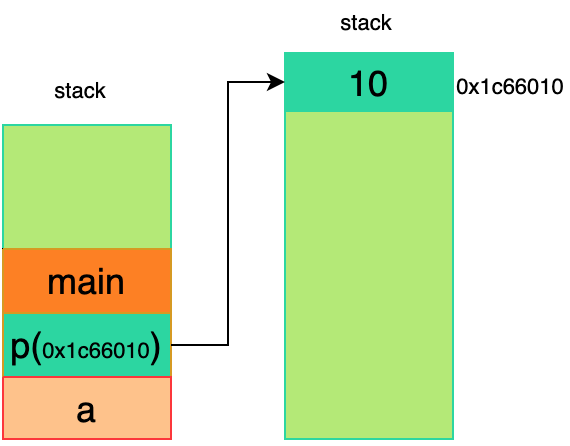## 产生方式

• 常发性内存泄漏
• 偶发性内存泄漏
• 一次性内存泄漏
• 隐式内存泄漏

### 偶发性内存泄漏

常发性内存泄漏不同的是，偶发性内存泄漏函数只在特定的场景下才会被执行。

### 一次性内存泄漏

int main() {
auto *service = new Service;
// do sth
service->Run();// 服务启动
service->Loop(); // 可以理解为一个sleep，目的是使得程序不退出
return 0;
}


### 隐式内存泄漏

• 内存碎片：还记得我们之前的那篇文章深入理解glibc内存管理精髓，程序跑了几天之后，进程就因为OOM导致了退出，就是因为内存碎片导致剩下的内存不能被重新分配导致
• 即使我们调用了free/delete，运行时库不一定会将内存归还OS，具体深入理解glibc内存管理精髓
• 用过STL的知道，STL内部有一个自己的allocator，我们可以当做一个memory poll，当调用vector.clear()时候，内存并不会归还OS，而是放回allocator，其内部根据一定的策略，在特定的时候将内存归还OS，是不是跟glibc原理很像😁

## 分类

### 未释放

int fun() {
char * pBuffer = malloc(sizeof(char));

/* Do some work */
return 0;
}


class Obj {
public:
Obj(int size) {
buffer_ = new char;
}
~Obj(){}
private:
char *buffer_;
};

int fun() {
Object obj;
// do sth
return 0;
}


~Object() {
delete buffer_;
}


### 未匹配

1. 通过operator new从堆上申请内存(glibc下，operator new底层调用的是malloc)
2. 调用构造函数(如果操作对象是一个class的话)

1. 调用对象的析构函数(如果操作对象是一个class的话)
2. 通过operator delete释放内存
void* operator new(std::size_t size) {
void* p = malloc(size);
if (p == nullptr) {
throw("new failed to allocate %zu bytes", size);
}
return p;
}
void* operator new[](std::size_t size) {
void* p = malloc(size);
if (p == nullptr) {
throw("new[] failed to allocate %zu bytes", size);
}
return p;
}

void  operator delete(void* ptr) throw() {
free(ptr);
}
void  operator delete[](void* ptr) throw() {
free(ptr);
}



class Test {
public:
Test() {
std::cout << "in Test" << std::endl;
}
// other
~Test() {
std::cout << "in ~Test" << std::endl;
}
};

int main() {
Test *t = new Test;
// do sth
delete t;
return 0;
}


1. 通过operator new申请内存(底层malloc实现)
2. 通过placement new在上述申请的内存块上调用构造函数
3. 调用ptr->~Test()释放Test对象的成员变量
4. 调用operator delete释放内存

// new
void *ptr = malloc(sizeof(Test));
t = new(ptr)Test

// delete
ptr->~Test();
free(ptr);


#### new 和 free

Test *t = new Test;
free(t)


#### new[] 和 delete

int main() {
Test *t = new Test ;
// do sth
delete t;
return 0;
}


in Test
in Test
in Test
in Test
in Test
in Test
in Test
in Test
in Test
in Test
in ~Test


### 虚析构

~basic_string() {
_M_rep()->_M_dispose(this->get_allocator());
}


typedef std::basic_string<char> string;


class Base {
public:
Base(){
buffer_ = new char;
}

~Base() {
std::cout << "in Base::~Base" << std::endl;
delete []buffer_;
}
private:
char *buffer_;

};

class Derived : public Base {
public:
Derived(){}

~Derived() {
std::cout << "int Derived::~Derived" << std::endl;
}
};

int main() {
Base *base = new Derived;
delete base;
return 0;
}


in Base::~Base


~Base() {
std::cout << "in Base::~Base" << std::endl;
delete []buffer_;
}


int Derived::~Derived
in Base::~Base


1. 调用父类的构造函数
2. 调用父类成员变量的构造函数
3. 调用派生类本身的构造函数

1. 执行派生类自身的析构函数
2. 执行派生类成员变量的析构函数
3. 执行父类的析构函数

### 循环引用

class Controller {
public:
Controller() = default;

~Controller() {
std::cout << "in ~Controller" << std::endl;
}

class SubController {
public:
SubController() = default;

~SubController() {
std::cout << "in ~SubController" << std::endl;
}

std::shared_ptr<Controller> controller_;
};

std::shared_ptr<SubController> sub_controller_;
};

int main() {
auto controller = std::make_shared<Controller>();
auto sub_controller = std::make_shared<Controller::SubController>();

controller->sub_controller_ = sub_controller;
sub_controller->controller_ = controller;
return 0;
}


int main() {
auto controller = std::make_shared<Controller>();
auto sub_controller = std::make_shared<Controller::SubController>();

controller->sub_controller_ = sub_controller;
sub_controller->controller_ = controller;

std::cout << "controller use_count: " << controller.use_count() << std::endl;
std::cout << "sub_controller use_count: " << sub_controller.use_count() << std::endl;
return 0;
}


controller use_count: 2
sub_controller use_count: 2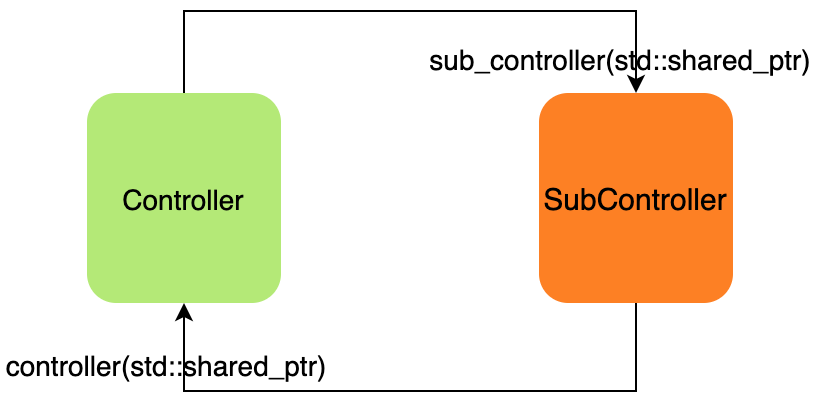class Controller {
public:
Controller() = default;

~Controller() {
std::cout << "in ~Controller" << std::endl;
}

class SubController {
public:
SubController() = default;

~SubController() {
std::cout << "in ~SubController" << std::endl;
}

std::weak_ptr<Controller> controller_;
};

std::shared_ptr<SubController> sub_controller_;
};


controller use_count: 1
sub_controller use_count: 2
in ~Controller
in ~SubController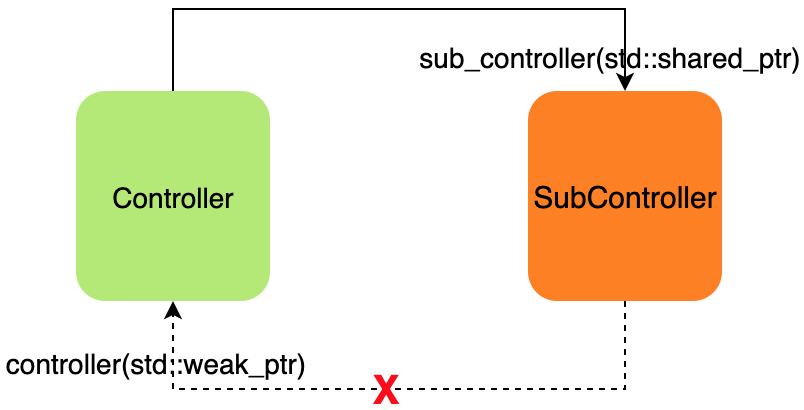std::shared_ptr controller = controller_.lock();


## 避免

### 手动释放

• 对于malloc函数分配的内存，在结束使用的时候，使用free函数进行释放
• 对于new操作符创建的对象，切记使用delete来进行释放
• 对于new []创建的对象，使用delete[]来进行释放(使用free或者delete均会造成内存泄漏)

### 避免使用裸指针

int fun(int *ptr) {// fun 是一个接口或lib函数
// do sth

return 0;
}

int main() {}
int a = 1000;
int *ptr = &a;
// ...
fun(ptr);

return 0;
}



### 使用STL中或者自己实现对象

• 使用std::string 替代char *, string类自己会进行内存管理，而且优化的相当不错
• 使用std::vector或者std::array来替代传统的数组
• 其它

### 智能指针

#### unique_ptr

unique_ptr是限制最严格的一种智能指针，用来替代之前的auto_ptr，独享被管理对象指针所有权。当unique_ptr对象被销毁时，会在其析构函数内删除关联的原始指针。

unique_ptr对象分为以下两类：

• unique_ptr 该类型的对象关联了单个Type类型的指针

std::unique_ptr<Type>   p1(new Type); // c++11
auto p1 = std::make_unique<Type>(); // c++14

• unique_ptr<Type[]> 该类型的对象关联了多个Type类型指针，即一个对象数组

std::unique_ptr<Type[]> p2(new Type[n]()); // c++11
auto p2 = std::make_unique<Type[]>(n); // c++14

• 不可用被复制

unique_ptr<int> a(new int(0));
unique_ptr<int> b = a;  // 编译错误
unique_ptr<int> b = std::move(a); // 可以通过move语义进行所有权转移


void fun() {
unique_ptr<int> a(new int(0));
// use a
}


#### shared_ptr

void fun() {
std::shared_ptr<Type> a; // a是一个空对象
{
std::shared_ptr<Type> b = std::make_shared<Type>(); // 分配资源
a = b; // 此时引用计数为2
{
std::shared_ptr<Type> c = a; // 此时引用计数为3
} // c退出作用域，此时引用计数为2
} // b 退出作用域，此时引用计数为1
} // a 退出作用域，引用计数为0，释放对象


#### weak_ptr

weak_ptr的出现，主要是为了解决shared_ptr的循环引用，其主要是与shared_ptr一起来私用。和shared_ptr不同的地方在于，其并不会拥有资源，也就是说不能访问对象所提供的成员函数，不过，可以通过weak_ptr.lock()来产生一个拥有访问权限的shared_ptr。

std::weak_ptr<Type> a;
{
std::shared_ptr<Type> b = std::make_shared<Type>();
a = b
} // b所对应的资源释放


### RAII

RAIIResource Acquisition is Initialization(资源获取即初始化)的缩写，是C++语言的一种管理资源，避免泄漏的用法。

RAII的做法是使用一个对象，在其构造时获取对应的资源，在对象生命周期内控制对资源的访问，使之始终保持有效，最后在对象析构的时候，释放构造时获取的资源。

std::mutex mutex_;

void fun() {
mutex_.lock();

if (...) {
mutex_.unlock();
return;
}

mutex_.unlock()
}


std::mutex mutex_;

void fun() {
std::lock_guard<std::mutex> guard(mutex_);

if (...) {
return;
}
}


## 定位

### 日志

char * fun() {
char *p = (char*)malloc(20);
printf("%s, %d, address is: %p", __FILE__, __LINE__, p);
// do sth
return p;
}

int main() {
fun();

return 0;
}


### 统计

static unsigned int allocated  = 0;
static unsigned int deallocated  = 0;
void *Memory_Allocate (size_t size)
{
void *ptr = NULL;
ptr = malloc(size);
if (NULL != ptr) {
++allocated;
} else {
//Log error
}
return ptr;
}
void Memory_Deallocate (void *ptr) {
if(pvHandle != NULL) {
free(ptr);
++deallocated;
}
}
int Check_Memory_Leak(void) {
int ret = 0;
if (allocated != deallocated) {
//Log error
ret = MEMORY_LEAK;
} else {
ret = OK;
}
return ret;
}


### 工具

#include <stdlib.h>

void func (void){
char *buff = (char*)malloc(10);
}

int main (void){
func(); // 产生内存泄漏
return 0;
}

• 通过gcc -g leak.c -o leak命令进行编译
• 执行valgrind --leak-check=full ./leak

==9652== Memcheck, a memory error detector
==9652== Copyright (C) 2002-2017, and GNU GPL'd, by Julian Seward et al.
==9652== Using Valgrind-3.15.0 and LibVEX; rerun with -h for copyright info
==9652== Command: ./leak
==9652==
==9652==
==9652== HEAP SUMMARY:
==9652==     in use at exit: 10 bytes in 1 blocks
==9652==   total heap usage: 1 allocs, 0 frees, 10 bytes allocated
==9652==
==9652== 10 bytes in 1 blocks are definitely lost in loss record 1 of 1
==9652==    at 0x4C29F73: malloc (vg_replace_malloc.c:309)
==9652==    by 0x40052E: func (leak.c:4)
==9652==    by 0x40053D: main (leak.c:8)
==9652==
==9652== LEAK SUMMARY:
==9652==    definitely lost: 10 bytes in 1 blocks
==9652==    indirectly lost: 0 bytes in 0 blocks
==9652==      possibly lost: 0 bytes in 0 blocks
==9652==    still reachable: 0 bytes in 0 blocks
==9652==         suppressed: 0 bytes in 0 blocks
==9652==
==9652== For lists of detected and suppressed errors, rerun with: -s
==9652== ERROR SUMMARY: 1 errors from 1 contexts (suppressed: 0 from 0)


valgrind的检测信息将内存泄漏分为如下几类：

• definitely lost：确定产生内存泄漏
• indirectly lost：间接产生内存泄漏
• possibly lost：可能存在内存泄漏
• still reachable：即使在程序结束时候，仍然有指针在指向该块内存，常见于全局变量

==9652==    by 0x40052E: func (leak.c:4)
==9652==    by 0x40053D: main (leak.c:8)


valgrind不仅可以检测内存泄漏，还有其他很强大的功能，由于本文以内存泄漏为主，所以其他的功能就不在此赘述了，有兴趣的可以通过valgrind --help来进行查看

### 经验之谈

• 良好的编程习惯，只有有malloc/new，就得有free/delete
• 尽可能的使用智能指针，智能指针就是为了解决内存泄漏而产生
• 使用log进行记录
• 也是最重要的一点，谁申请，谁释放

## 结语

C/C++之所以复杂、效率高，是因为其灵活性，可用直接访问操作系统API，而正因为其灵活性，就很容易出问题，团队成员必须愿意按照一定的规则来进行开发，有完整的review机制，将问题暴露在上线之前。这样才可以把经历放在业务本身，而不是查找这些问题上，有时候往往一个小问题就能消耗很久的时间去定位解决，所以，一定要有一个良好的开发习惯posted @ 2022-01-13 06:06  高性能架构探索  阅读(2018)  评论(16编辑  收藏  举报